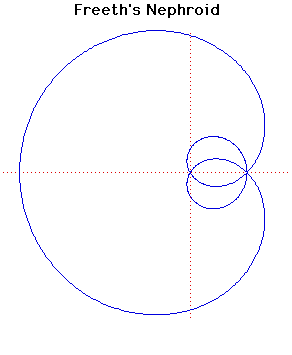# Curves

### Freeth's NephroidPolar equation:
$r = a(1 + 2\sin( \theta /2))$

### Description

This is a strophoid of a circle with the pole $O$ at the centre of the circle and the fixed point $P$ on the circumference of the circle.

In the picture above, $O$ is the origin and $P$ is the node where the curve crosses itself three times.

If the line through $P$ parallel to the $y$-axis cuts the nephroid at $A$ then angle $AOP$ is 3π /7 . This can be used to construct a regular 7 sided figure.

T J Freeth (1819-1904) was an English mathematician. In a paper published by the London Mathematical Society in 1879 he described various strophoids, including the strophoid of a trisectrix.

### Associated Curves

Definitions of the Associated curves